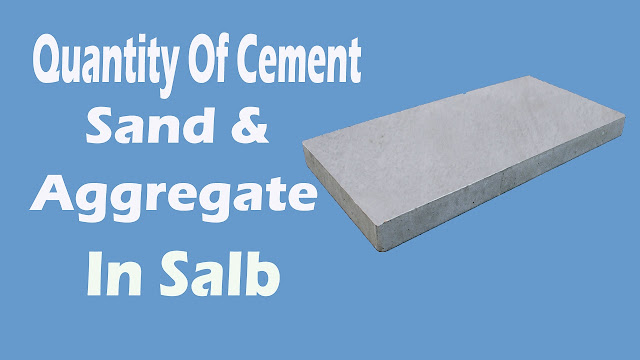How to Calculate Quantity for Cement , Sand and Aggregate in Slab

# How to Calculate Quantity for Cement , Sand and Aggregate in SlabIn this video you will learn Step by Step How to Calculate Quantity for Cement , Sand & Aggregate.Quantities of materials for concrete such as cement, sand and aggregates for production of required quantity of concrete of given mix proportions such as 1:2:4 (M15), 1:1.5: 3 (M20), 1:1:2 (M25) can be calculated by absolute volume method.

This method is based on the principle that the volume of fully compacted concrete is equal to the absolute volume of all the materials of concrete, i.e. cement, sand, coarse aggregates and water.

A Concrete structure may consists of beams, slabs, columns and foundations etc. based on type of structure. The volume of concrete required for concrete structure can be calculated by summing up the volumes of each structural member or each parts of members.

The volume of a rectangular cross sectional member can be calculated as length x width x height (or depth or thickness). Suitable formula shall be used for different cross-sectional shapes of members.

Watch the video...

Continue Reading about the "How to Calculate Quantity for Cement , Sand and Aggregate in Slab" on the next page below